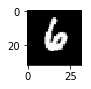# Lenet to recognizance handwritten numbers

Load MNIST  dataset comes pre-loaded with TensorFlow all you have to do is the following commands

from tensorflow.examples.tutorials.mnist import input_data

X_train, y_train           = mnist.train.images, mnist.train.labels
X_validation, y_validation = mnist.validation.images, mnist.validation.labels
X_test, y_test             = mnist.test.images, mnist.test.labels

assert(len(X_train) == len(y_train))
assert(len(X_validation) == len(y_validation))
assert(len(X_test) == len(y_test))

print("\n Image Shape: {} \n".format(X_train[].shape))

These dataset has been previously split into sets of 55000 samples with image shape: (28, 28, 1).
NOTE: Is good practice to use assert to confirm that all the data has been loaded correctly.
To find out how large the training set you can run:print("Training Set: {} samples".format(len(X_train)))

### 2. Prepare Data Set

In many cases, this step requires data augmentation and more. Because MNIST dataset has a 28x28x1 shape and the architecture we are using for this example only accepts 32x32x? images (? = color channels), we are just going to reshape images to 1x32x32x1

import numpy as np
img_size = 32

if X_train[].shape is not img_size:

print("Updated Image Shape: {}".format(X_train[].shape))


Updated Image Shape: (32, 32, 1)

if I have and array = [1, 2, 3, 4, 5]
>>> np.lib.pad(array, (2,3), 'constant', constant=(4, 6))
array([4, 4, 1, 2, 3, 4, 5, 6, 6, 6])

### 3. Take a look at the data set, How does this dataset look like?

import random
import matplotlib.pyplot as plt
%matplotlib inline

index = random.randint(, len(X_train))
image = X_train[index].squeeze()

plt.figure(figsize=(1,1))
plt.imshow(image, cmap="gray")
print(y_train[index])### 3. Architecture

For this example, I am using LeNet architecture http://yann.lecun.com/exdb/publis/pdf/lecun-01a.pdf

import tensorflow as tf
from tensorflow.contrib.layers import flatten

tf.reset_default_graph()

# Using tf.truncated_normal to initilize weights and biases varibles
# with random normal distribution
mu =
sigma = 0.1

conv1_W = tf.Variable(tf.truncated_normal(shape=(5, 5, 1, 6), mean = mu, stddev = sigma))
conv1_b = tf.Variable(tf.zeros(6))

conv2_W = tf.Variable(tf.truncated_normal(shape=(5, 5, 6, 16), mean = mu, stddev = sigma))
conv2_b = tf.Variable(tf.zeros(16))

fc1_W = tf.Variable(tf.truncated_normal(shape=(400, 120), mean = mu, stddev = sigma))
fc1_b = tf.Variable(tf.zeros(120))

fc2_W  = tf.Variable(tf.truncated_normal(shape=(120, 84), mean = mu, stddev = sigma))
fc2_b  = tf.Variable(tf.zeros(84))

fc3_W  = tf.Variable(tf.truncated_normal(shape=(84, 10), mean = mu, stddev = sigma))
fc3_b  = tf.Variable(tf.zeros(10))

def LeNet(x):

print(x.shape)
#Layer 1: Convolutional. Input = 32x32x1. Output = 28x28x6.
conv1   = tf.nn.conv2d(x, conv1_W, strides=[1, 1, 1, 1], padding='VALID') + conv1_b
conv1 = tf.nn.relu(conv1)
#Pooling. Input = 28x28x6. Output = 14x14x6.
conv1 = tf.nn.max_pool(conv1, ksize=[1, 2, 2, 1], strides=[1, 2, 2, 1], padding='VALID')

#Layer 2: Convolutional. Output = 10x10x16.
conv2   = tf.nn.conv2d(conv1, conv2_W, strides=[1, 1, 1, 1], padding='VALID') + conv2_b
conv2 = tf.nn.relu(conv2)
#Pooling. Input = 10x10x16. Output = 5x5x16.
conv2 = tf.nn.max_pool(conv2, ksize=[1, 2, 2, 1], strides=[1, 2, 2, 1], padding='VALID')
#Flatten. Input = 5x5x16. Output = 400.
fc0   = flatten(conv2)

#Layer 3: Fully Connected. Input = 400. Output = 120.
fc1   = tf.matmul(fc0, fc1_W) + fc1_b
fc1    = tf.nn.relu(fc1)

#Layer 4: Fully Connected. Input = 120. Output = 84.
fc2    = tf.matmul(fc1, fc2_W) + fc2_b
fc2    = tf.nn.relu(fc2)

#Layer 5: Fully Connected. Input = 84. Output = 10.
logits = tf.matmul(fc2, fc3_W) + fc3_b

return logits

#Placeholder variables
x = tf.placeholder(tf.float32, (None, img_size, img_size, 1))
y = tf.placeholder(tf.int32, (None))

save_path = 'model/model.ckpt'

placeholder is a variable that we can use to transfer a value when we run our Tensorflow session.
It creates a memory space for variables that will be using in the future.
In this case x stands for our images and y for our labels

<strong>Logits</strong> is a matrix with an estimate number of how likely the input image is to be of the a class. In order for this number to look like a provability we have to normilize them (zero to one) using softmax.

In the following section, you would notice that we would be using BATCH_SIZE = 128. This means that instead of feeding images in training data one by one to the network we would be feeing 128 at one time and let the GPU/CPU process all of them in parallel. and compute loss, calculate the gradient and update weights and proceed with next image. The choice of this size is up to you and depends on your problem too.

### 4. Deep learning pipeline Model

EPOCHS = 10
BATCH_SIZE = 128

rate = 0.001
one_hot_y = tf.one_hot(y, 10)

# Get logits
logits = LeNet(x)
# Computes softmax cross entropy between logits and labels
cross_entropy = tf.nn.softmax_cross_entropy_with_logits(labels=one_hot_y, logits=logits)
#Calculate loss
loss_operation = tf.reduce_mean(cross_entropy)
training_operation = optimizer.minimize(loss_operation)

# Add an op to initialize the variables.
init_op = tf.global_variables_initializer()
saver = tf.train.Saver()



### 5. Set a validation system

correct_prediction = tf.equal(tf.argmax(logits, 1), tf.argmax(one_hot_y, 1))
accuracy_operation = tf.reduce_mean(tf.cast(correct_prediction, tf.float32))

def evaluate(X_data, y_data):
num_examples = len(X_data)
total_accuracy =
sess = tf.get_default_session()
for offset in range(, num_examples, BATCH_SIZE):
batch_x, batch_y = X_data[offset:offset+BATCH_SIZE], y_data[offset:offset+BATCH_SIZE]
accuracy = sess.run(accuracy_operation, feed_dict={x: batch_x, y: batch_y})
total_accuracy += (accuracy * len(batch_x))


### 6. Start Tensorflow training session

import time
beginTime = time.time()
with tf.Session() as sess:
sess.run(init_op)
dataLen = len(X_train)

print("Training...")
print()
for i in range(EPOCHS):
for offset in range(, dataLen, BATCH_SIZE):
end = offset + BATCH_SIZE
batch_x, batch_y = X_train[offset:end], y_train[offset:end]
sess.run(training_operation, feed_dict={x: batch_x, y: batch_y})

validation_accuracy = evaluate(X_validation, y_validation)

endTime = time.time()
print("{:5.2f}s EPOCH {} Accuracy = {:.3f} \n".format(\
endTime - beginTime, i+1, validation_accuracy))

save_path = saver.save(sess, 'model/model.ckpt')
print("Model saved")


Training...

4.94s EPOCH 1 Accuracy = 0.958

7.64s EPOCH 2 Accuracy = 0.970

10.23s EPOCH 3 Accuracy = 0.975

12.85s EPOCH 4 Accuracy = 0.978

15.48s EPOCH 5 Accuracy = 0.982

18.06s EPOCH 6 Accuracy = 0.982

20.69s EPOCH 7 Accuracy = 0.984

23.31s EPOCH 8 Accuracy = 0.983

25.92s EPOCH 9 Accuracy = 0.987

28.55s EPOCH 10 Accuracy = 0.987

Model saved## Manuel Cuevas

Hello, I'm Manuel Cuevas a Software Engineer with background in machine learning and artificial intelligence.